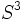# Group actions on topological spaces of cyclic group:Z2

This article describes various actions of the cyclic group of order two on topological spaces. Two actions of a group on a topological space are equivalent if one can be conjugated to another by a homeomorphism of the topological space.

Of particular interest are properly discontinuous group actions. Since the group is finite, properly discontinuous in this context simply means an action without any fixed points. More specifically, a properly discontinuous group action of the cyclic group of order two is equivalent to a fixed-point free self-homeomorphism of the topological space, whose square is the identity map.

Properly discontinuous group actions of the cyclic group of order two, on a connected orientable manifold, can be classified into two types:

1. Those where the action of the non-identity element is orientation-preserving: In this case, the quotient space is orientable. In fact, specifying an orientation on the original manifold automatically specifies an orientation on the quotient.
2. Those where the action of the non-identity element is orientation-reversing: In this case, the quotient space is non-orientable.

## Action on spheres

### Antipodal action on spheres (properly discontinuous)

The typical example of a properly discontinuous action of the cyclic group of order two is the antipodal action on the sphere. Here, the non-identity element of the cyclic group sends each point on the sphere$S^n$, to its antipode, or diametrically opposite point. The quotient of$S^n$ by this action is real projective space, denoted$\R\mathbb{P}^n$.

When$n$ is odd, this action is orientation-preserving, and thus, real projective space of odd dimension is orientable.

When$n$ is even, this action is orientation-reversing, so real projective space of odd dimension is non-orientable.

### Other actions on spheres

An interesting problem is the classification, upto equivalence, of all properly discontinuous group actions on spheres.

## Actions on compact connected orientable surfaces

### Properly discontinuous actions

The compact connected orientable surface of genus$g$ is a surface with$g$ holes. The cyclic group of order two has an orientation-preserving properly discontinuous action on a surface of genus$g$ if and only if$g$ is odd.

The proof that the genus needs to be odd follows from a general Euler characteristic computation, using the fact that if the action is orientation-preserving, the quotient is also orientable, and then using the fact that the Euler characteristic of the new surface is half that of the old surface.

For odd$g$, an explicit action can be visualized as follows: arrange the holes such that there is one central hole, and there are$g-1$ holes whose centers are in a circle about the central hole, arranged like the vertices of a regular polygon. Since$g-1$ is even, a rotation by$\pi$ sends this figure to itself, and has no fixed points.

### Other actions

If we do not require the action to be properly discontinuous, we can get many more actions, including on surfaces of even genus. For instance, the surface of genus two has a homeomorphism of order two that flips the two holes -- however, this homeomorphism has a fixed-point set of dimension either zero or one.

## Actions on Lie groups

Given a Lie group, there are two special sources of properly discontinuous group actions on it, namely:

1. Actions via left or right multiplication by elements of order two: Left and right multiplications are always orientation-preserving, so the quotients are always orientable.
2. Actions via smooth group automorphisms of order two

### Actions via multiplication

Suppose$G$ is a connected Lie group and$x \in G$ is a non-identity element of order two. Then, the two-element subgroup generated by$x$ acts properly discontinuously on$G$ by left multiplication. The quotient space is, set-theoretically, the same as the coset space of$G$ by this two-element subgroup. In the case that the two-element subgroup is normal (or, equivalently, central, because any discrete normal subgroup in a connected Lie group is central), the quotient is actually a Lie group.

Here are some examples:

1. We can quotient out the circle group$S^1$ by the unique subgroup of order two. We get the group$\R\mathbb{P}^1$, that is also isomorphic to$S^1$.
2. We can quotient out the group$S^3$ by the subgroup of order two, to get$\R\mathbb{P}^3 \cong SO(3)$.